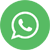ANOVA Video Tutorial

## Description

ANOVA stands for analysis of variance which is a hypothesis testing technique. Before we discuss about ANOVA, let us discuss about the equation Y = f(X). Y is called as output variable & X is called as input variable. If Y is of continuous data type & if X is also continuous & if we have more than two Xs then we go ahead with a technique called ANOVA. Hypothesis Testing in general is used to determine on whether a decision should be taken or not based on the given business scenario.

ANOVA will help us evaluate multiple continuous Xs. For e.g., if you want to evaluate the performance of 3 machines based on turn around time then we are effectively comparing machines A, B, C based on turn around time. Under these kind of circumstances we use ANOVA. Once again we have one-way ANOVA & two-way ANOVA based on how many levels we use to compare the different groups. For example, if we are evaluating the performance of employees based on various departments alone then it is one-way ANOVA. If we evaluate performance of employees based on departments & also shifts (day, night) then it is two-way ANOVA. If we use more than 2 levels to evaluate different scenarios then we can use MANOVA.